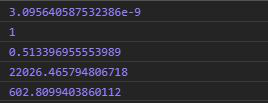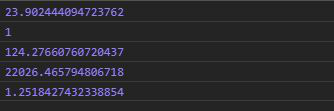# D3.js randomLogNormal() Function

• Last Updated : 03 Aug, 2020

The d3.randomLogNormal() function is used to generate the random number based on the log-normal function.

Syntax:

Hey geek! The constant emerging technologies in the world of web development always keeps the excitement for this subject through the roof. But before you tackle the big projects, we suggest you start by learning the basics. Kickstart your web development journey by learning JS concepts with our JavaScript Course. Now at it's lowest price ever!

```d3.randomLogNormal(mu,sigma);
```

Parameters: This function accepts two parameters as mentioned above and described below:

• mu: It is the expected value of the random variable.
• sigma: The number is generated with a given standard deviation called sigma.

Returns: It returns a function.

Note: If mu and sigma is not given then mu is equal to 0 and sigma to 1 by default.

Below given are a few examples of the above function.

Example 1: The output may be different each time the function is executed.

## HTML

 ```<``html` `lang``=``"en"``>``<``head``>``  ``<``meta` `charset``=``"UTF-8"``>``  ``<``meta` `name``=``"viewport"` `        ``content``=``"width=device-width, initial-scale=1.0"``>``  ``<``title``>Document````<``style``>````<``body``>``  ````  ``<``script` `type` `= ``"text/javascript"` `          ``src` `= ``"https://d3js.org/d3.v4.min.js"``>``  ````  ``<``script``>``    ``// Output may be different each time ``    ``// the function is run``    ``console.log(d3.randomLogNormal(2,10)())``    ``console.log(d3.randomLogNormal(0,0)())``    ``console.log(d3.randomLogNormal(1,12)())``    ``console.log(d3.randomLogNormal(10,0)())``    ``console.log(d3.randomLogNormal(5,1)())``  ```````

Output:Example 2:

## HTML

 ```<``html` `lang``=``"en"``>``<``head``>``  ``<``meta` `charset``=``"UTF-8"``>``  ``<``meta` `name``=``"viewport"` `        ``content``=``"width=device-width, initial-scale=1.0"``>``  ``<``title``>Document````<``style``>````<``body``>``  ````  ``<``script` `type` `= ``"text/javascript"` `          ``src` `= ``"https://d3js.org/d3.v4.min.js"``>``  ````  ``<``script``>``    ``// Output may be different each time ``    ``// the function is run``    ``console.log(d3.randomLogNormal(2,10)(1))``    ``console.log(d3.randomLogNormal(0,0)(2))``    ``console.log(d3.randomLogNormal(1,12)(3))``    ``console.log(d3.randomLogNormal(10,0)(0))``    ``// When mu and sigma is not given``    ``console.log(d3.randomLogNormal()())``  ```````

Output:My Personal Notes arrow_drop_up